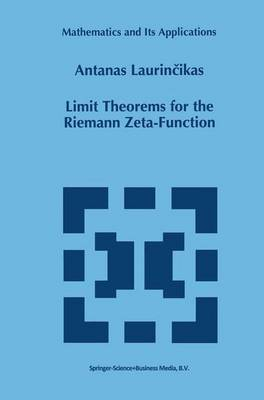•# Limit Theorems for the Riemann Zeta-Function - Mathematics and Its Applications 352 (Hardback)

(author)
£119.99
Hardback 306 Pages
Published: 30/11/1995
• We can order this from the publisher

UK delivery within 3-4 weeks

The subject of this book is probabilistic number theory. In a wide sense probabilistic number theory is part of the analytic number theory, where the methods and ideas of probability theory are used to study the distribution of values of arithmetic objects. This is usually complicated, as it is difficult to say anything about their concrete values. This is why the following problem is usually investigated: given some set, how often do values of an arithmetic object get into this set? It turns out that this frequency follows strict mathematical laws. Here we discover an analogy with quantum mechanics where it is impossible to describe the chaotic behaviour of one particle, but that large numbers of particles obey statistical laws. The objects of investigation of this book are Dirichlet series, and, as the title shows, the main attention is devoted to the Riemann zeta-function. In studying the distribution of values of Dirichlet series the weak convergence of probability measures on different spaces (one of the principle asymptotic probability theory methods) is used. The application of this method was launched by H. Bohr in the third decade of this century and it was implemented in his works together with B. Jessen. Further development of this idea was made in the papers of B. Jessen and A. Wintner, V. Borchsenius and B.

Publisher: Springer
ISBN: 9780792338246
Number of pages: 306
Weight: 1390 g
Dimensions: 234 x 156 x 19 mm
Edition: 1996 ed.